# Temperature Measurement & Real-Time Display

Two Particle Photons are used to power a temperature sensor and OLED display. The temp sensor records the ambient temp at 30 sec intervals.

IntermediateFull instructions provided6 hours567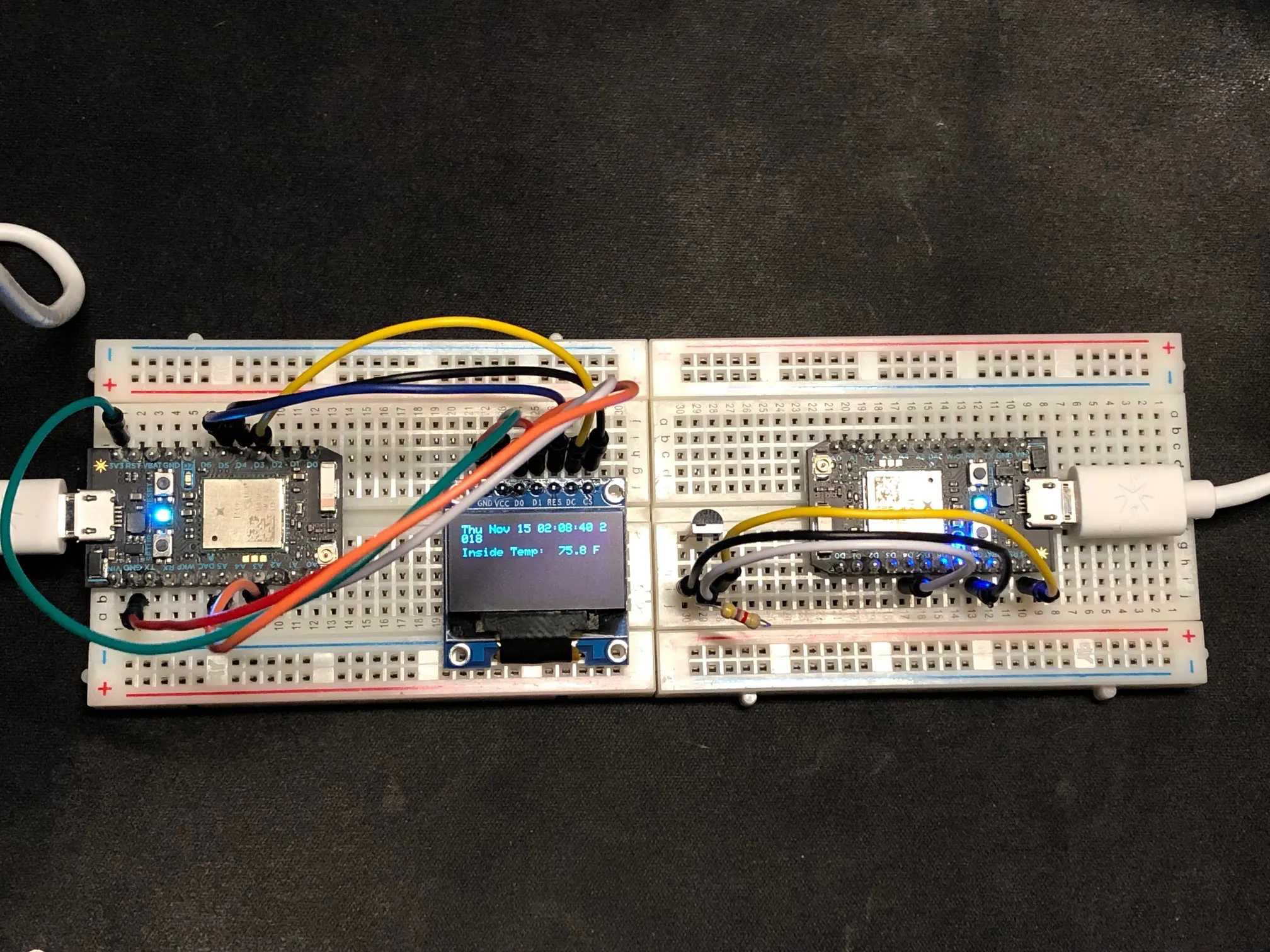## Things used in this project

### Hardware componentsParticle Photon
×2
 Male/Male Jumper Wires
×10
×1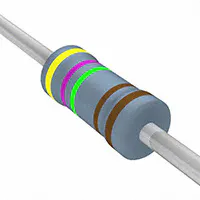Resistor 4.75k ohm
×1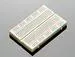×2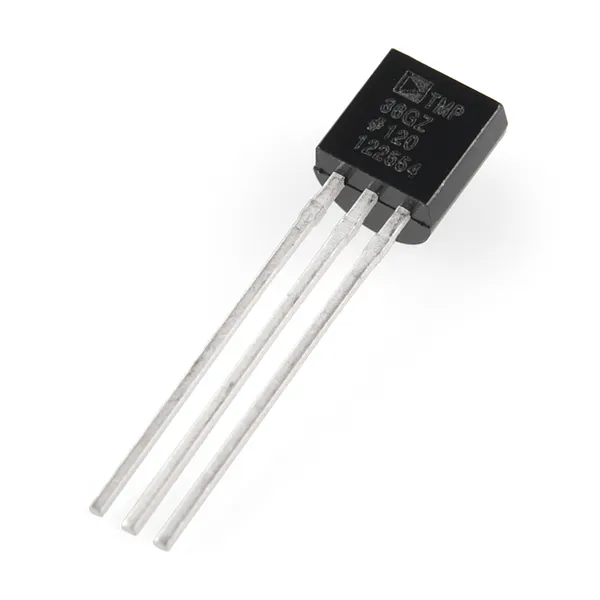Temperature Sensor
×1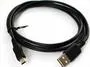USB-A to Mini-USB Cable
×2

### Software apps and online servicesIFTTT Maker service## Schematics

### Temperature Sensor

This is the schematic for the photon and temperature circuit.

### Temperature Sensor### OLED Display

This is the schematic for the photon and Adafruit OLED display.

### OLED Display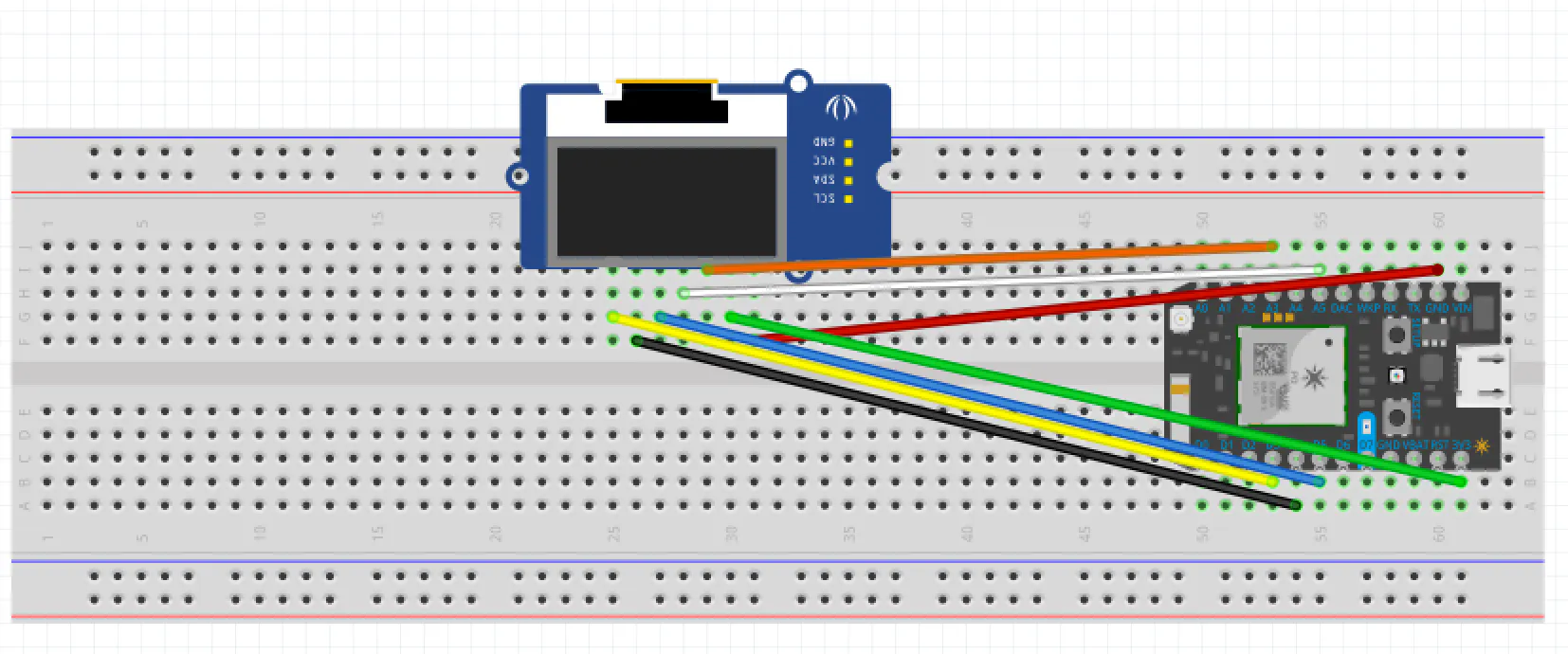### Transient Temperature Sensor Performance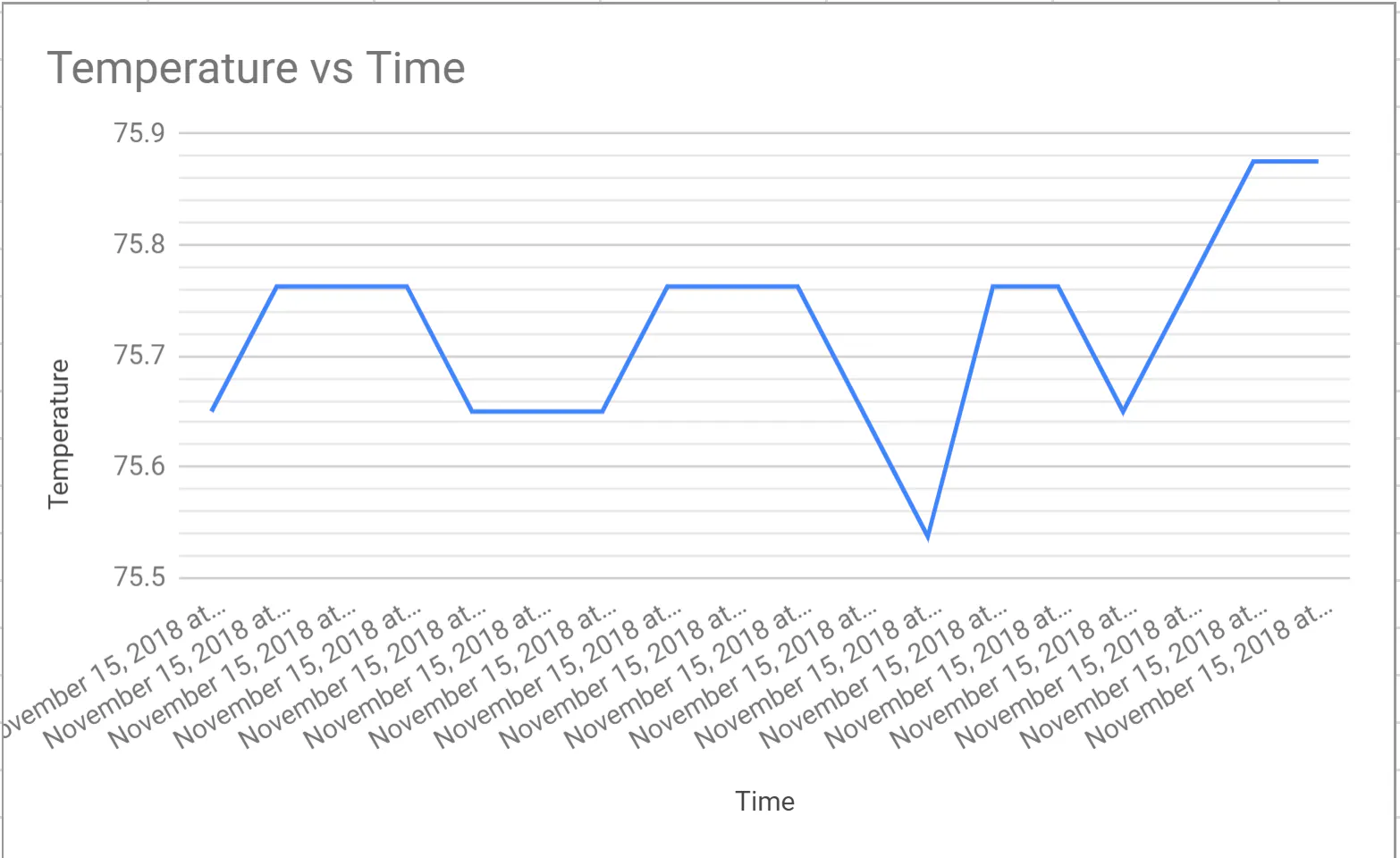## Code

### Temperature Sensor

C/C++
This code controls the temperature sensor and the photon paired with it. Note that the code contains the libray "OneWire.h".
```// This #include statement was automatically added by the Particle IDE.
#include <OneWire.h>

OneWire ds = OneWire(D4);  // 1-wire signal on pin D4

unsigned long lastUpdate = 0;

float lastTemp;

void setup() {
Serial.begin(9600);
// Set up 'power' pins, comment out if not used!
pinMode(D3, OUTPUT);
pinMode(D5, OUTPUT);
digitalWrite(D3, LOW);
digitalWrite(D5, HIGH);
pinMode(D7,OUTPUT);
}

void myHandler(const char *event, const char *data){
if (strcmp(data, "1")==0){
digitalWrite(D7, HIGH);
delay(1000);
digitalWrite(D7, LOW);
}
}
// up to here, it is the same as the address acanner
// we need a few more variables for this example

void loop(void) {
byte i;
byte present = 0;
byte type_s;
byte data;
float celsius, fahrenheit;

Serial.println();
ds.reset_search();
delay(250);
return;
}

// The order is changed a bit in this example
// first the returned address is printed

Serial.print("ROM =");
for( i = 0; i < 8; i++) {
Serial.write(' ');
}

// second the CRC is checked, on fail,

Serial.println("CRC is not valid!");
return;
}
Serial.println();

// we have a good address at this point
// what kind of chip do we have?
// we will set a type_s value for known types or just return

// the first ROM byte indicates which chip
case 0x10:
Serial.println("  Chip = DS1820/DS18S20");
type_s = 1;
break;
case 0x28:
Serial.println("  Chip = DS18B20");
type_s = 0;
break;
case 0x22:
Serial.println("  Chip = DS1822");
type_s = 0;
break;
case 0x26:
Serial.println("  Chip = DS2438");
type_s = 2;
break;
default:
Serial.println("Unknown device type.");
return;
}

// this device has temp so let's read it

ds.reset();               // first clear the 1-wire bus
ds.select(addr);          // now select the device we just found
// ds.write(0x44, 1);     // tell it to start a conversion, with parasite power on at the end
ds.write(0x44, 0);        // or start conversion in powered mode (bus finishes low)

// just wait a second while the conversion takes place
// different chips have different conversion times, check the specs, 1 sec is worse case + 250ms
// you could also communicate with other devices if you like but you would need

delay(1000);     // maybe 750ms is enough, maybe not, wait 1 sec for conversion

// we might do a ds.depower() (parasite) here, but the reset will take care of it.

// first make sure current values are in the scratch pad

present = ds.reset();
ds.write(0xB8,0);         // Recall Memory 0
ds.write(0x00,0);         // Recall Memory 0

present = ds.reset();
if (type_s == 2) {
ds.write(0x00,0);       // The DS2438 needs a page# to read
}

// transfer and print the values

Serial.print("  Data = ");
Serial.print(present, HEX);
Serial.print(" ");
for ( i = 0; i < 9; i++) {           // we need 9 bytes
Serial.print(data[i], HEX);
Serial.print(" ");
}
Serial.print(" CRC=");
Serial.print(OneWire::crc8(data, 8), HEX);
Serial.println();

// Convert the data to actual temperature
// because the result is a 16 bit signed integer, it should
// be stored to an "int16_t" type, which is always 16 bits
// even when compiled on a 32 bit processor.
int16_t raw = (data << 8) | data;
if (type_s == 2) raw = (data << 8) | data;
byte cfg = (data & 0x60);

switch (type_s) {
case 1:
raw = raw << 3; // 9 bit resolution default
if (data == 0x10) {
// "count remain" gives full 12 bit resolution
raw = (raw & 0xFFF0) + 12 - data;
}
celsius = (float)raw * 0.0625;
break;
case 0:
// at lower res, the low bits are undefined, so let's zero them
if (cfg == 0x00) raw = raw & ~7;  // 9 bit resolution, 93.75 ms
if (cfg == 0x20) raw = raw & ~3; // 10 bit res, 187.5 ms
if (cfg == 0x40) raw = raw & ~1; // 11 bit res, 375 ms
// default is 12 bit resolution, 750 ms conversion time
celsius = (float)raw * 0.0625;
break;

case 2:
data = (data >> 3) & 0x1f;
if (data > 127) {
celsius = (float)data - ((float)data * .03125);
}else{
celsius = (float)data + ((float)data * .03125);
}
}

// remove random errors
if((((celsius <= 0 && celsius > -1) && lastTemp > 5)) || celsius > 125) {
celsius = lastTemp;
}

fahrenheit = celsius * 1.8 + 32.0;
lastTemp = celsius;
Serial.print("  Temperature = ");
Serial.print(celsius);
Serial.print(" Celsius, ");
Serial.print(fahrenheit);
Serial.println(" Fahrenheit");

// now that we have the readings, we can publish them to the cloud
String temperature = String(fahrenheit); // store temp in "temperature" string
Particle.publish("Xtemperature/3171_MEGR", temperature); // publish to cloud
delay(30000); // 30 second delay //every 1 second is "1000"
}
```

### OLED Display Code

C/C++
This code powers the Adafruit SSD1306 OLED display and the accompanying photon. Note that the code contains the library "Adafruit_SSD1306.h".
```// This #include statement was automatically added by the Particle IDE.

//OLED Screen code:

#define OLED_DC     D3
#define OLED_CS     D4
#define OLED_RESET  D5

char temp;
String tempO;

int ledon = 1;
int flashd7 = 0;
void setup()
{

Particle.subscribe("Xtemperature/3171_MEGR", myHandler);

display.begin(SSD1306_SWITCHCAPVCC);
display.clearDisplay();
display.display();

}

void loop()
{

display.clearDisplay();
display.setTextColor(WHITE);
display.setTextSize(1);
display.setCursor(0,0);
Time.zone(-4);
display.print(Time.timeStr().c_str());
display.setCursor(0,20);
display.printf("Inside Temp:  %0.4s F", temp);
display.display();
delay(5000);
if(flashd7 == 1) {
flashd7 = 0;
}
}

void myHandler(const char *event, const char *data)
{
flashd7 = 1;
strcpy(temp,data);

}
```

## Credits

### Joseph Lam

1 project • 0 followers

### Charlton Buckler

1 project • 0 followers